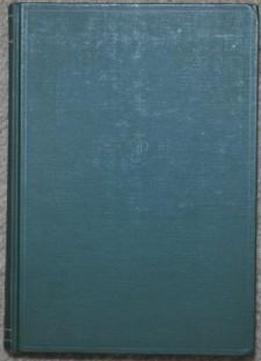Number Theory (pure & Applied Mathematics) by Igor R. Shafarevich / 1966 / English / PDF

Modern number theory, according to Hecke, dates from Gauss's quadratic reciprocity law. The various extensions of this law and the generalizations of the domains of study for number theory have led to a rich network of ideas, which has had effects throughout mathematics, in particular in algebra. This volume of the Encyclopaedia presents the main structures and results of algebraic number theory with emphasis on algebraic number fields and class field theory. Koch has written for the non-specialist. He assumes that the reader has a general understanding of modern algebra and elementary number theory. Mostly only the general properties of algebraic number fields and related structures are included. Special results appear only as examples which illustrate general features of the theory. A part of algebraic number theory serves as a basic science for other parts of mathematics, such as arithmetic algebraic geometry and the theory of modular forms. For this reason, the chapters on basic number theory, class field theory and Galois cohomology contain more detail than the others. This book is suitable for graduate students and research mathematicians who wish to become acquainted with the main ideas and methods of algebraic number theory.

Modern number theory, according to Hecke, dates from Gauss's quadratic reciprocity law. The various extensions of this law and the generalizations of the domains of study for number theory have led to a rich network of ideas, which has had effects throughout mathematics, in particular in algebra. This volume of the Encyclopaedia presents the main structures and results of algebraic number theory with emphasis on algebraic number fields and class field theory. Koch has written for the non-specialist. He assumes that the reader has a general understanding of modern algebra and elementary number theory. Mostly only the general properties of algebraic number fields and related structures are included. Special results appear only as examples which illustrate general features of the theory. A part of algebraic number theory serves as a basic science for other parts of mathematics, such as arithmetic algebraic geometry and the theory of modular forms. For this reason, the chapters on basic number theory, class field theory and Galois cohomology contain more detail than the others. This book is suitable for graduate students and research mathematicians who wish to become acquainted with the main ideas and methods of algebraic number theory.

views: 677